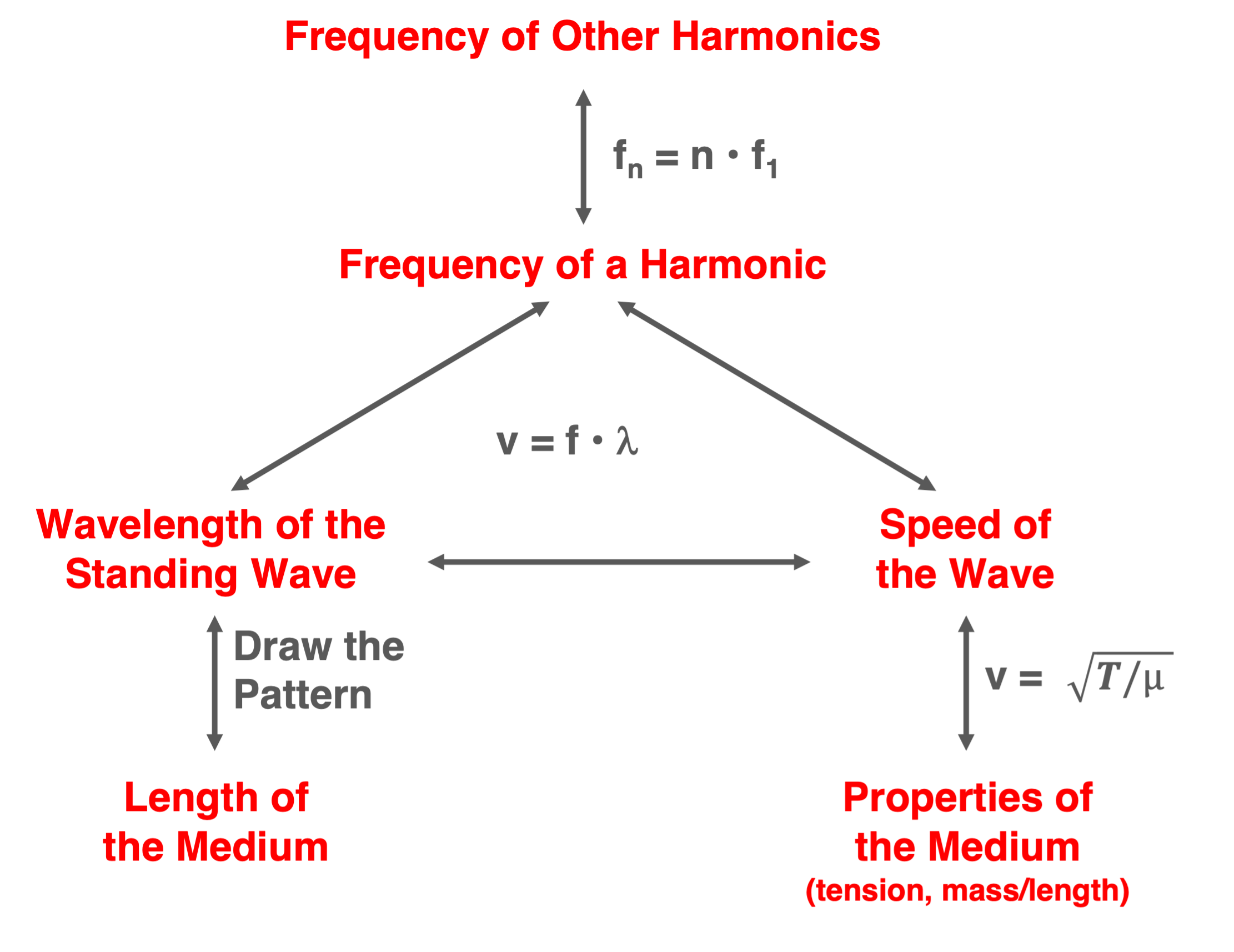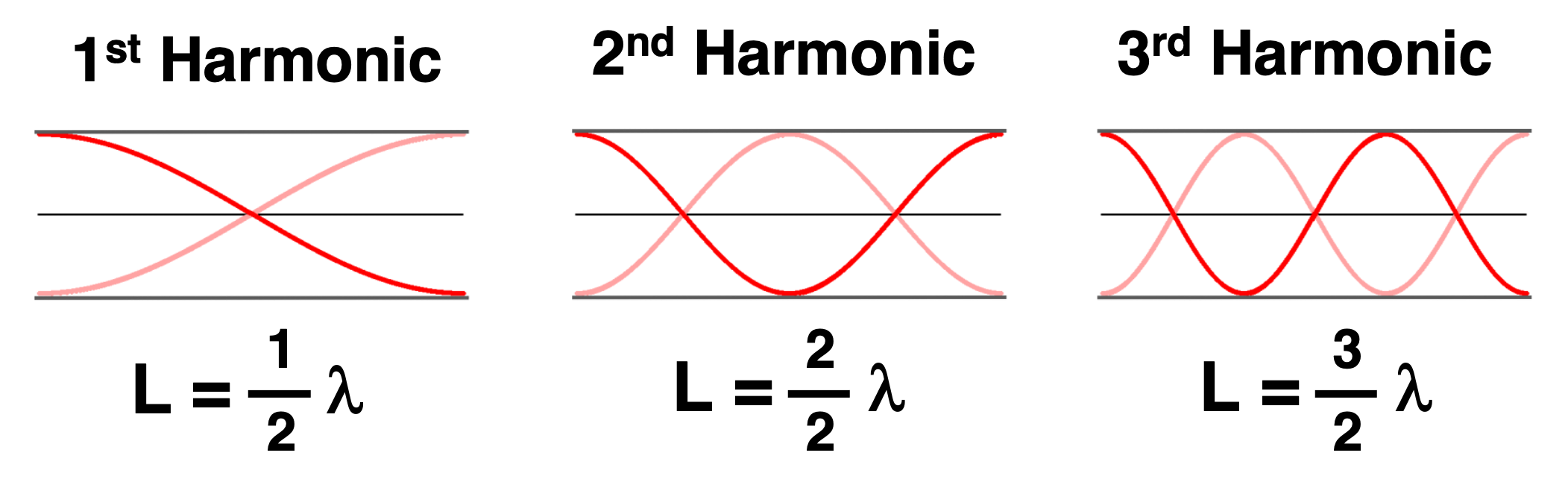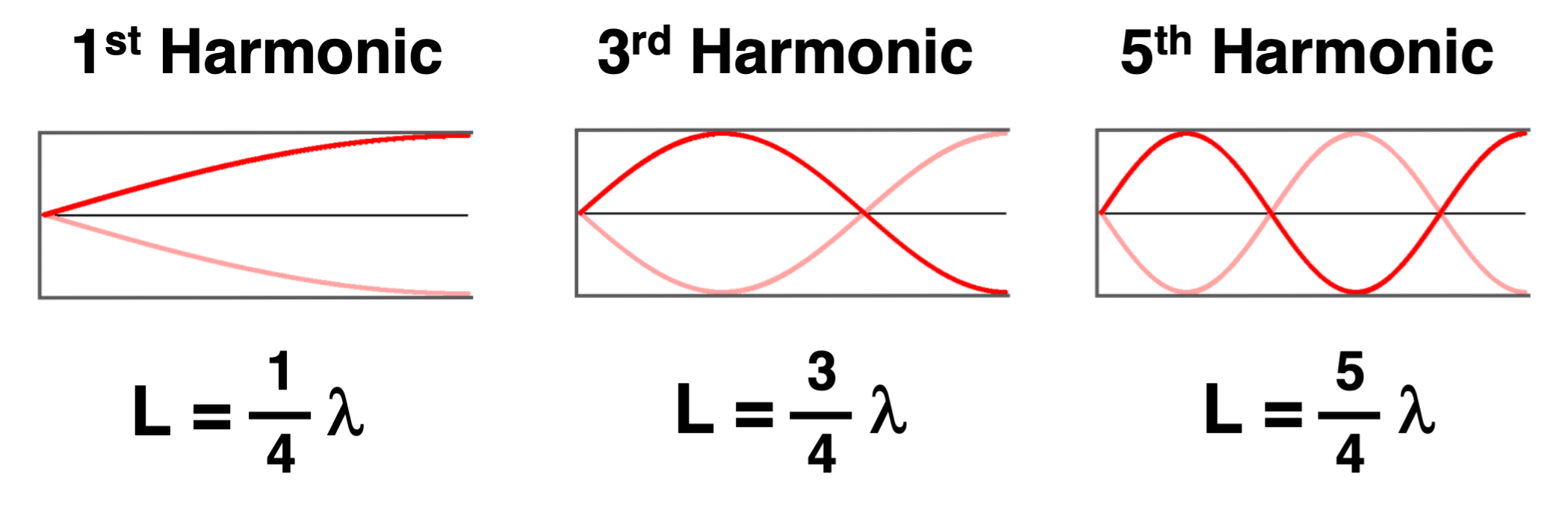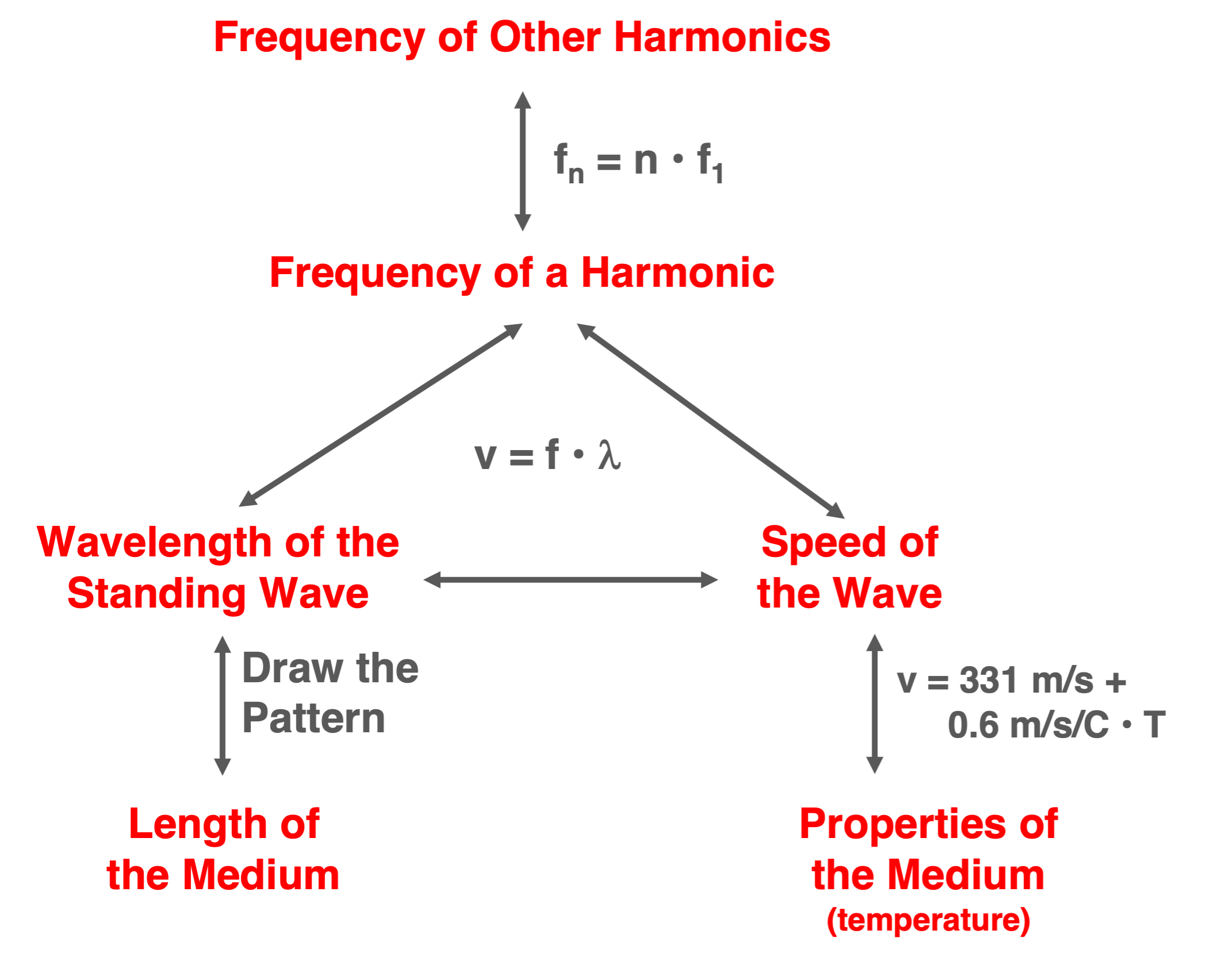# Waves, Sound and Light: Sound Waves

## Sound Waves: Equation Overview

There are 23 ready-to-use problem sets on the topic of Sound Waves.  The problems target your ability to determine wave quantities such as frequency, period, wavelength, intensity and speed from verbal descriptions and diagrams of physical situations pertaining to sound waves and resonance in strings and air columns. Problems range in difficulty from the very easy and straight-forward to the very difficult and complex.

## The Speed of Sound

The speed of any wave (v) is defined as the distance traveled (d) per time of travel (t) and is described by the following equation:

v = d / t

Thus, the distance traveled by a wave is related to the time required for it to travel that distance.

The speed of sound, like the speed of any wave, is dependent upon the properties of the medium through which it is moving. For a sound wave moving through air, the primary property of air that effects its speed is the temperature of the air. There are a couple of equations that describe the speed (v) - temperature (T) relationship; the following might be the easiest to remember:

v = 331 m/s + (0.6 m/s/°C) •T

where T is the Celsius temperature of the air through which the sound wave is moving. At 0°C, the speed of sound is 331 m/s. For every degree Celsius above 0°C, the speed of sound increases by approximately 0.6 m/s. This equation provides a rather accurate estimate of the speed of sound for temperatures upwards towards 50°C.

## Sound Intensity

Sound waves are produced by a vibrating object - vocal chords, guitar string, or diaphragm on a speaker. The sound waves begin at a point (or approximately a point) and then propagate through space in three dimensions. As the sound wave propagates, it creates a wave front that fills the surface area of an ever-expanding sphere. A sound wave (like any wave) is often referred to as an energy-transport phenomenon. The rate at which energy is put into the wave is referred to as the power of the source. Power (P) is expressed in units of Watts. As these vibrations propagate through space, they become less intense as the size of the spherical wave front expands. Whatever energy is created by the wave at the source fills the surface area of a sphere some distance R away. Because the sphere is constantly expanding, the energy is becoming diluted with increasing distance from the source. The sound intensity (I) at any given location is defined as the rate at which energy arrives at that location. Because the wave energy is spreading over a surface area, intensity is often expressed in units of Watts/meter2 and given by the equation

I = P / (4 • π • R2)

You might recognize the 4•π•R2 as being the surface area of a sphere having a radius of R.

## The deciBel Scale

The human ear is sensitive enough to detect the smallest of vibrations. The lowest amplitude vibration which most humans can hear is defined as the threshold of hearing (TOH). While such a sound will be different for different people, the intensity associated with this sound is defined as 1.0 x 10-12 W/m2. The range of sound intensities that a typical human can detect is enormous. Sound which 100 billion times more intense than the threshold of hearing (1 x 10-1 W/m2) is typically detectable without pain. Intensities beyond this level begin to cause pain and possibly the risk of hearing loss. Because there is an enormous range of intensities from the threshold of hearing to the threshold of pain, a logarithmic scale - known as the decibel scale - is often used to express sound intensity. This deciBel scale simply expresses the intensity level of any sound in terms of how many factors of 10 greater its intensity is compared to the threshold of hearing (1 x 10-12 W/m2). A sound which is 10 times more intense than the TOH is 1 bel. A sound which is 100 (102) times more intense than the TOH is 2 bels. And a sound which is 1000 (103) times more intense than the TOH is 3 bels. More commonly, the sound level is expressed in the smaller unit decibel (1/10-th of a Bel), abbreviated dB. A sound rated at 1 bel is a 10 deciBel sound; a sound rated at 2 bel is a 20 deciBel sound; and so forth.

The deciBel level can be properly determined from the intensity (I) of a sound using the following equation:

dB = 10 • log ( I / 1.0 x 10-12 W/m2 )

Often times, the deciBel level is known and the intensity in W/m2 is desired. In such instances the equation below can be used.

I = 1.0 x 10-12 W/m2 • 10x where x = dB/10

Decibel levels decrease as the distance from the source of sound increases. This is due to the intensity-distance relationship discussed in the previous section. As the distance from the source doubles, the intensity decreases by a factor of 4 (22). As distance from the source triples, the intensity decreases by a factor of 9 (32). And as distance from the source quadruples, the intensity decreases by a factor of 16 (42). This is known as the inverse square relationship between intensity and distance. But since the deciBel level is not linearly related to the intensity, the same inverse square relationship does not exist for deciBel level and distance. So if given the deciBel level at one location and asked to determine the deciBel level at another location, it is important to approach the problem in three steps.

1. Calculate the intensity at the first location.
2. Apply the inverse square law to determine the intensity at the second location.
3. Calculate the deciBel level at the second location using the intensity value at that location.

(It is worth noting that there are short-cuts to this three step method that is dependent upon an undestanding of logarathmic relationships. For those confident with logarithms, we invite you to give the short-cut a try ... but do make an effort to understand the physics of the inverse square law.)

## The Doppler Effect

As a source of sound moves toward or away from an observer, the pitch of the sound is different than the actual source frequency. This phenomenon is known as the Doppler effect. As the source of sound approaches you, there is an upward shift in pitch or frequency relative to the source frequency. And as the source of sound moves away from you, there is a downward shift in frequency relative to the source frequency. This phenomenon is often noticed as an ambulance with its siren on passes by you as you are parked along the roadside. The pitch is higher as the ambulance approaches and noticeably lower after it has passed and is moving away from you. The frequency (f') that is heard can be calculated by using the Doppler Shift equations.

f' = f / (1 ± vs/v)

where v = the speed of sound, vs = the speed of the source of sound, f = the frequency of the source, and f' = observed frequency. In the denominator of this equation, the minus sign is used for situations in which the source is approaching the observer and the plus sign is used in situations in which the source is moving away from the observer.

The same phenomenon would be observed when the observer is approaching a stationary source or moving away from the stationary source. Once more, the frequency that is heard can be calculated by using the Doppler Shift equations.

f' = (1 ± vo/v)•f

where v = the speed of sound, vo = the speed of the observer, f = the frequency of the source, and f' = observed frequency. In this equation, the plus sign is used for situations in which the observer is approaching the source and the minus sign is used in situations in which the observer is moving away from the source.

## Speed of Waves in Strings, Ropes, Wires and Cables

The speed of a wave depends upon the properties of the medium through which it is transmitted, and NOT upon the properties of the wave itself. For sound waves being transmitted through strings, wires, ropes and cables, the primary properties effecting wave speed are the tension of the medium and the mass density of the medium. Tension pertains to the force with which the two ends of the medium are pulled tight. Being a force, it is expressed with the unit Newton. Mass density pertains to the mass per unit length of the string, wire, rope or cable and is expressed in standard units of kilogram/meter (kg/m). The equation expressing the relationship between these variables is

v = √(T/μ)

where v represents the wave speed, T represents the tension, and μ represents the mass density. When using these equations, it is important to pay attention to the units with which the given quantities are expressed and to make appropriate conversions where necessary. It is recommended that substitutions be made into the equation using standard metric units. For speed, use m/s; for tension, use Newtons (abbreviated N); and for mass density, use kg/m.

## Frequency-Wavelength-Speed Relationship

Many of the questions in this unittarget your ability to analyze physical situations involving the wavelength-frequency-speed relationship for standing wave patterns in strings, wires, ropes, cables and air columns. Any wave, whether a standing wave or a traveling wave, will have a wavelength-frequency-speed relationship which follows the equation:

v = f • λ

where v represents the speed (or velocity) of the wave, f represents the frequency of the wave, and λ represents the wavelength of the wave. As mentioned above, the speed of a wave is dependent upon the properties of the medium. For strings, wires, ropes and cables, the properties of importance are the tension and mass density. For air columns, the property of importance is the temperature of the air. As such, the properties of the medium are also related to the frequency and the wavelength of the wave.

## Resonance in Vibrating Strings, Ropes, Wires and Cables

Waves introduced into a string, wire, rope or cable will typically travel the length of the medium and reflect back upon reaching its end. At certain frequencies, the reflected portion of the wave meets up with the original wave to create a pattern known as a standing wave pattern. In a standing wave pattern there are points along the medium that appear as if they are always standing still. These points are known as nodes and are easily remembered as the points of no desplacement (properly spelled as displacement). Separating the nodes are anti-nodes: points of maximum positive and negative displacement. In such standing wave patterns, there is a unique half-number relationship between the length of the medium and the wavelength of the waves that have established the pattern seen. These relationships are shown below for the standing wave patterns having one anti-node (first harmonic), two anti- nodes (second harmonic) and three anti- nodes (third harmonic).It is clear from the above graphic that the length of the string, wire, rope or cable is related to the wavelength of the standing wave that is established within it. As such, the length of the medium is related mathematically to the frequency of the wave and the speed of the waver (or the properties of the medium upon which wave speed depends).

A standing wave pattern such as those shown in the graphic above is established within a medium only when it is being disturbed at specific frequencies. Not any frequency will result in a standing wave pattern; only the discrete frequencies that lead to wavelength values that are mathematically related to the length of the medium as illustrated by these equations. Such frequencies are known as the harmonic frequencies of the string, wire, rope or cable. As observed in the graphic, the pattern associated with the second harmonic has a wavelength that is one-half the wavelength of the pattern associated with the first harmonic. And the pattern associated with the third harmonic has a wavelength that is one-third the wavelength of the pattern associated with the first harmonic. Continuing this same logic, one would reason, that the pattern associated with the fifth harmonic has a wavelength which is one-fifth the wavelength of the pattern associated with the first harmonic. And in general, the pattern associated with the nth harmonic has a wavelength that is 1 / n the wavelength of the pattern associated with the first harmonic. Each harmonic pattern - whether the second, third, fifth or nth - is characterized by a wavelength which is smaller than the wavelength of the first by a factor of n. In this case, n is known as the harmonic number.

λn = λ1 / n

The speeds of the waves for each of these harmonics is the same. Thus, the decrease in wavelength which results from a progression from the first to the third (and higher) harmonic must correspond to an increase in the frequency by the same factor n. That is, the frequency of the second harmonic is two times the frequency of the first harmonic; the frequency of the third harmonic is three times the frequency of the first harmonic; and the frequency of the nth harmonic is n times the frequency of the first harmonic. Put in equation form, one could state that

fn = n • f1

where fn is the frequency of any harmonic pattern, n is the harmonic number associated with that pattern and f1 is the frequency of the first harmonic. The frequency of the first harmonic (f1) is the fundamental frequency; it is the lowest possible frequency at which a standing wave could be established within the medium.

## An Effective Problem-Solving Strategy for Resonating Strings

Solving problems relating to resonating strings targets a student's ability to relate the frequency, wavelength and speed of waves to properties of the string and to the length of the string. The graphic below depicts the relationships between the various quantities in such problems. As is the usual case, when approaching a problem, first identify what you know and what you are trying to find. Locate the knowns and unknowns on the graphic below and plot out a strategy that allows you to determine the unknown quantity. The strategy for solving for the unknown will be centered around the relationships depicted in the graphic. The stated equations provide the mathematical expression of those relationships.## Resonance in Air Columns

As just mentioned, a standing wave can be established in a string when vibrating at one of its resonance frequencies. Similarly, a column of air can resonate as well, provided that another object vibrating at one of the resonance frequencies forces the air into vibration. The resonance vibrations of an air column is the basis of many of the later problems in this unit. The air column is either open at both ends (open-end air column) or open at one end and closed at the other (closed-end air column). A resonance situation in an open-end air column is characterized by the presence of a vibrational anti-nodes at each of the open ends, creating the standing wave patterns shown below. Each pattern is referred to as a harmonic and has its own unique wavelength and frequency. As shown in the graphic, there is a distinct relationship between the wavelength of the standing wave and the length of the air column. Knowing the pattern allows one to relate the length to the wavelength and ultimately to the frequency and the speed.A closed-end air column is open to the surrounding air at one end and closed at the other end. A resonance situation in a closed-end air column is characterized by the presence of a vibrational anti-node at the open end and a vibrational node at the closed end, creating the standing wave patterns shown below. Again, there is a distinct relationship between the length of the air columns and the wavelength for each of the harmonics. Knowing this length-wavelength relationship allows one to relate the length of an air column to the speed and the frequency at which the air inside naturally vibrates.## An Effective Problem-Solving Strategy for Resonating Air Columns

A strategy for solving problems related to resonating strings was discussed above. A similar strategy can be applied in the approach to problems involving resonating air columns. Such problems target a student's ability to relate the frequency, wavelength and speed of waves to properties of the air column (temperature) and to the length of the air column. The graphic below depicts the relationships between the various quantities in such problems. As is the usual case, when approaching a problem, first identify what you know and what you are trying to find. Locate the knowns and unknowns on the graphic below and plot out a strategy which allows you to determine the unknown quantity. The strategy for solving for the unknown will be centered around the relationships depicted in the graphic. The stated equations provide the mathematical expression of those relationships.## Interference and Beats

When two sound sources of very similar yet different frequencies meet at an observer's ears the phenomenon of beats is observed. This phenomenon is perceived by an observer as a sound that fluctuates in amplitude very rapidly over the course of time. For instance, if two tuning forks - one with a frequency of 256 Hz and the other with a frequency of 254 Hz - produce sound waves, then an observer would hear a fluctuation in amplitude at a frequency of 2 Hz. This 2 Hz fluctuation in frequency is known as the beat frequency and is equivalent to the absolute value of the difference in frequency between the two sources.

Beat Frequency = | f2 - f1 |

The fluctuations in amplitude which are observed is the result of the interference of the two waves. A low amplitude sound is observed when a compression from one source meets up with a rarefaction from the other source. A high amplitude sound is observed when a compression from one source meets up with a compression from the other source (or a rarefaction with a rarefaction).

## Habits of an Effective Problem-Solver

An effective problem solver by habit approaches a physics problem in a manner that reflects a collection of disciplined habits. While not every effective problem solver employs the same approach, they all have habits which they share in common. These habits are described briefly here. An effective problem-solver...

• ...reads the problem carefully and develops a mental picture of the physical situation. If needed, they sketch a simple diagram of the physical situation to help visualize it.
• ...identifies the known and unknown quantities, often times recording them on the diagram itself. They equate given values to the symbols used to represent the corresponding quantity (e.g., v = 345 m/s, λ = 1.28 m, f = ???).
• ...plots a strategy for solving for the unknown quantity; the strategy will typically center around the use of physics equations and be heavily dependent upon an understanding of physics principles.
• ...identifies the appropriate formula(s) to use, often times writing them down. Where needed, they perform the needed conversion of quantities into the proper unit.
• ...performs substitutions and algebraic manipulations in order to solve for the unknown quantity.